# 15.2: Entropy Rules

$$\newcommand{\vecs}{\overset { \rightharpoonup} {\mathbf{#1}} }$$ $$\newcommand{\vecd}{\overset{-\!-\!\rightharpoonup}{\vphantom{a}\smash {#1}}}$$$$\newcommand{\id}{\mathrm{id}}$$ $$\newcommand{\Span}{\mathrm{span}}$$ $$\newcommand{\kernel}{\mathrm{null}\,}$$ $$\newcommand{\range}{\mathrm{range}\,}$$ $$\newcommand{\RealPart}{\mathrm{Re}}$$ $$\newcommand{\ImaginaryPart}{\mathrm{Im}}$$ $$\newcommand{\Argument}{\mathrm{Arg}}$$ $$\newcommand{\norm}{\| #1 \|}$$ $$\newcommand{\inner}{\langle #1, #2 \rangle}$$ $$\newcommand{\Span}{\mathrm{span}}$$ $$\newcommand{\id}{\mathrm{id}}$$ $$\newcommand{\Span}{\mathrm{span}}$$ $$\newcommand{\kernel}{\mathrm{null}\,}$$ $$\newcommand{\range}{\mathrm{range}\,}$$ $$\newcommand{\RealPart}{\mathrm{Re}}$$ $$\newcommand{\ImaginaryPart}{\mathrm{Im}}$$ $$\newcommand{\Argument}{\mathrm{Arg}}$$ $$\newcommand{\norm}{\| #1 \|}$$ $$\newcommand{\inner}{\langle #1, #2 \rangle}$$ $$\newcommand{\Span}{\mathrm{span}}$$$$\newcommand{\AA}{\unicode[.8,0]{x212B}}$$

##### Learning Objectives

You are expected to be able to define and explain the significance of terms identified in bold.

• A reversible process is one carried out in infinitessimal steps after which, when undone, both the system and surroundings (that is, the world) remain unchanged (see the example of gas expansion-compression below). Although true reversible change cannot be realized in practice, it can always be approximated.
• ((in which a process is carried out.
• As a process is carried out in a more reversible manner, the value of w approaches its maximum possible value, and q approaches its minimum possible value.
• Although q is not a state function, the quotient qrev/T is, and is known as the entropy.
• energy within a system.
• The entropy of a substance increases with its molecular weight and complexity and with temperature. The entropy also increases as the pressure or concentration becomes smaller. Entropies of gases are much larger than those of condensed phases.
• The absolute entropy of a pure substance at a given temperature is the sum of all the entropy it would acquire on warming from absolute zero (where S=0) to the particular temperature.

Entropy is one of the most fundamental concepts of physical science, with far-reaching consequences ranging from cosmology to chemistry. It is also widely mis-represented as a measure of "disorder", as we discuss below. The German physicist Rudolf Clausius originated the concept as "energy gone to waste" in the early 1850s, and its definition went through a number of more precise definitions over the next 15 years.

Previously, we explained how the tendency of thermal energy to disperse as widely as possible is what drives all spontaneous processes, including, of course chemical reactions. We now need to understand how the direction and extent of the spreading and sharing of energy can be related to measurable thermodynamic properties of substances— that is, of reactants and products.

You will recall that when a quantity of heat q flows from a warmer body to a cooler one, permitting the available thermal energy to spread into and populate more microstates, that the ratio q/T measures the extent of this energy spreading. It turns out that we can generalize this to other processes as well, but there is a difficulty with using q because it is not a state function; that is, its value is dependent on the pathway or manner in which a process is carried out. This means, of course, that the quotient q/T cannot be a state function either, so we are unable to use it to get differences between reactants and products as we do with the other state functions. The way around this is to restrict our consideration to a special class of pathways that are described as reversible.

## Reversible and irreversible changes

A change is said to occur reversibly when it can be carried out in a series of infinitesimal steps, each one of which can be undone by making a similarly minute change to the conditions that bring the change about. For example, the reversible expansion of a gas can be achieved by reducing the external pressure in a series of infinitesimal steps; reversing any step will restore the system and the surroundings to their previous state. Similarly, heat can be transferred reversibly between two bodies by changing the temperature difference between them in infinitesimal steps each of which can be undone by reversing the temperature difference.

The most widely cited example of an irreversible change is the free expansion of a gas into a vacuum. Although the system can always be restored to its original state by recompressing the gas, this would require that the surroundings perform work on the gas. Since the gas does no work on the surrounding in a free expansion (the external pressure is zero, so PΔV = 0,) there will be a permanent change in the surroundings. Another example of irreversible change is the conversion of mechanical work into frictional heat; there is no way, by reversing the motion of a weight along a surface, that the heat released due to friction can be restored to the system.Figure $$\PageIndex{1}$$: Reversible vs. Irreversible Expansions and Compressions

These diagrams show the same expansion and compression ±ΔV carried out in different numbers of steps ranging from a single step at the top to an "infinite" number of steps at the bottom. As the number of steps increases, the processes become less irreversible; that is, the difference between the work done in expansion and that required to re-compress the gas diminishes. In the limit of an ”infinite” number of steps (bottom), these work terms are identical, and both the system and surroundings (the “world”) are unchanged by the expansion-compression cycle. In all other cases the system (the gas) is restored to its initial state, but the surroundings are forever changed.

##### Definition: Reversible Changes

A reversible change is one carried out in such as way that, when undone, both the system and surroundings (that is, the world) remain unchanged.

It should go without saying, of course, that any process that proceeds in infinitesimal steps would take infinitely long to occur, so thermodynamic reversibility is an idealization that is never achieved in real processes, except when the system is already at equilibrium, in which case no change will occur anyway! So why is the concept of a reversible process so important?

The answer can be seen by recalling that the change in the internal energy that characterizes any process can be distributed in an infinity of ways between heat flow across the boundaries of the system and work done on or by the system, as expressed by the First Law ΔU = q + w. Each combination of q and w represents a different pathway between the initial and final states. It can be shown that as a process such as the expansion of a gas is carried out in successively longer series of smaller steps, the absolute value of q approaches a minimum, and that of w approaches a maximum that is characteristic of the particular process.

Thus when a process is carried out reversibly, the w-term in the First Law expression has its greatest possible value, and the q-term is at its smallest. These special quantities wmax and qmin (which we denote as qrev and pronounce “q-reversible”) have unique values for any given process and are therefore state functions.

### Work and reversibility

For a process that reversibly exchanges a quantity of heat qrev with the surroundings, the entropy change is defined as

$\Delta S = \dfrac{q_{rev}}{T} \label{23.2.1}$

This is the basic way of evaluating ΔS for constant-temperature processes such as phase changes, or the isothermal expansion of a gas. For processes in which the temperature is not constant such as heating or cooling of a substance, the equation must be integrated over the required temperature range, as discussed below.Figure $$\PageIndex{2}$$: Note that the reversible condition implies wmax and qmin. The impossibility of extracting all of the internal energy as work is essentially a statement of the Second Law.

If no real process can take place reversibly, what use is an expression involving qrev? This is a rather fine point that you should understand: although transfer of heat between the system and surroundings is impossible to achieve in a truly reversible manner, this idealized pathway is only crucial for the definition of ΔS; by virtue of its being a state function, the same value of ΔS will apply when the system undergoes the same net change via any pathway. For example, the entropy change a gas undergoes when its volume is doubled at constant temperature will be the same regardless of whether the expansion is carried out in 1000 tiny steps (as reversible as patience is likely to allow) or by a single-step (as irreversible a pathway as you can get!) expansion into a vacuum.

## The physical meaning of entropy

Entropy is a measure of the degree of spreading and sharing of thermal energy within a system. This “spreading and sharing” can be spreading of the thermal energy into a larger volume of space or its sharing amongst previously inaccessible microstates of the system. The following table shows how this concept applies to a number of common processes.

Table $$\PageIndex{1}$$: Observations and Explanations in terms of Entropy changes
system and process source of entropy increase of system
A deck of cards is shuffled, or 100 coins, initially heads up, are randomly tossed. This has nothing to do with entropy because macro objects are unable to exchange thermal energy with the surroundings within the time scale of the process
Two identical blocks of copper, one at 20°C and the other at 40°C, are placed in contact. The cooler block contains more unoccupied microstates, so heat flows from the warmer block until equal numbers of microstates are populated in the two blocks.
A gas expands isothermally to twice its initial volume. A constant amount of thermal energy spreads over a larger volume of space
1 mole of water is heated by 1C°. The increased thermal energy makes additional microstates accessible. (The increase is by a factor of about
1020,000,000,000,000, 000,000,000.)
Equal volumes of two gases are allowed to mix. The effect is the same as allowing each gas to expand to twice its volume; the thermal energy in each is now spread over a larger volume.
One mole of dihydrogen, H2, is placed in a container and heated to 3000K. Some of the H2 dissociates to H because at this temperature there are more thermally accessible microstates in the 2 moles of H.
The above reaction mixture is cooled to 300K. The composition shifts back to virtually all H2because this molecule contains more thermally accessible microstates at low temperatures.

Entropy is an extensive quantity; that is, it is proportional to the quantity of matter in a system; thus 100 g of metallic copper has twice the entropy of 50 g at the same temperature. This makes sense because the larger piece of copper contains twice as many quantized energy levels able to contain the thermal energy.

Entropy and "disorder"

Entropy is still described, particularly in older textbooks, as a measure of disorder. In a narrow technical sense this is correct, since the spreading and sharing of thermal energy does have the effect of randomizing the disposition of thermal energy within a system. But to simply equate entropy with “disorder” without further qualification is extremely misleading because it is far too easy to forget that entropy (and thermodynamics in general) applies only to molecular-level systems capable of exchanging thermal energy with the surroundings. Carrying these concepts over to macro systems may yield compelling analogies, but it is no longer science. it is far better to avoid the term “disorder” altogether in discussing entropy.### Entropy and Probability

The distribution of thermal energy in a system is characterized by the number of quantized microstates that are accessible (i.e., among which energy can be shared); the more of these there are, the greater the entropy of the system. This is the basis of an alternative (and more fundamental) definition of entropy

$\color{red} S = k \ln Ω \label{23.2.2}$

in which k is the Boltzmann constant (the gas constant per molecule, 1.3810–23 J K–1) and Ω (omega) is the number of microstates that correspond to a given macrostate of the system. The more such microstates, the greater is the probability of the system being in the corresponding macrostate. For any physically realizable macrostate, the quantity Ω is an unimaginably large number, typically around $$10^{10^{25}}$$ for one mole. By comparison, the number of atoms that make up the earth is about $$10^{50}$$. But even though it is beyond human comprehension to compare numbers that seem to verge on infinity, the thermal energy contained in actual physical systems manages to discover the largest of these quantities with no difficulty at all, quickly settling in to the most probable macrostate for a given set of conditions.

The reason S depends on the logarithm of Ω is easy to understand. Suppose we have two systems (containers of gas, say) with S1, Ω1 and S2, Ω2. If we now redefine this as a single system (without actually mixing the two gases), then the entropy of the new system will be

$S = S_1 + S_2$

but the number of microstates will be the product Ω1Ω2because for each state of system 1, system 2 can be in any of Ω2 states. Because

$\ln(Ω_1Ω_2) = \ln Ω_1 + \ln Ω_2$

Hence, the additivity of the entropy is preserved.

If someone could make a movie showing the motions of individual atoms of a gas or of a chemical reaction system in its equilibrium state, there is no way you could determine, on watching it, whether the film is playing in the forward or reverse direction. Physicists describe this by saying that such systems possess time-reversal symmetry; neither classical nor quantum mechanics offers any clue to the direction of time.

However, when a movie showing changes at the macroscopic level is being played backward, the weirdness is starkly apparent to anyone; if you see books flying off of a table top or tea being sucked back up into a tea bag (or a chemical reaction running in reverse), you will immediately know that something is wrong. At this level, time clearly has a direction, and it is often noted that because the entropy of the world as a whole always increases and never decreases, it is entropy that gives time its direction. It is for this reason that entropy is sometimes referred to as "time's arrow".

But there is a problem here: conventional thermodynamics is able to define entropy change only for reversible processes which, as we know, take infinitely long to perform. So we are faced with the apparent paradox that thermodynamics, which deals only with differences between states and not the journeys between them, is unable to describe the very process of change by which we are aware of the flow of time.

The direction of time is revealed to the chemist by the progress of a reaction toward its state of equilibrium; once equilibrium is reached, the net change that leads to it ceases, and from the standpoint of that particular system, the flow of time stops. If we extend the same idea to the much larger system of the world as a whole, this leads to the concept of the "heat death of the universe" that was mentioned briefly in the previous lesson.

## Absolute Entropies

Energy values, as you know, are all relative, and must be defined on a scale that is completely arbitrary; there is no such thing as the absolute energy of a substance, so we can arbitrarily define the enthalpy or internal energy of an element in its most stable form at 298K and 1 atm pressure as zero. The same is not true of the entropy; since entropy is a measure of the “dilution” of thermal energy, it follows that the less thermal energy available to spread through a system (that is, the lower the temperature), the smaller will be its entropy. In other words, as the absolute temperature of a substance approaches zero, so does its entropy. This principle is the basis of the Third law of thermodynamics, which states that the entropy of a perfectly-ordered solid at 0 K is zero.

##### Third law of thermodynamics

The entropy of a perfectly-ordered solid at 0 K is zero.

The absolute entropy of a substance at any temperature above 0 K must be determined by calculating the increments of heat q required to bring the substance from 0 K to the temperature of interest, and then summing the ratios q/T. Two kinds of experimental measurements are needed:

1. The enthalpies associated with any phase changes the substance may undergo within the temperature range of interest. Melting of a solid and vaporization of a liquid correspond to sizeable increases in the number of microstates available to accept thermal energy, so as these processes occur, energy will flow into a system, filling these new microstates to the extent required to maintain a constant temperature (the freezing or boiling point); these inflows of thermal energy correspond to the heats of fusion and vaporization. The entropy increase associated with melting, for example, is just ΔHfusion/Tm.
2. The heat capacity C of a phase expresses the quantity of heat required to change the temperature by a small amount ΔT , or more precisely, by an infinitesimal amount dT . Thus the entropy increase brought about by warming a substance over a range of temperatures that does not encompass a phase transition is given by the sum of the quantities C dT/T for each increment of temperature dT . This is of course just the integral

$S_{0^o \rightarrow T^o} = \int _{o^o}^{T^o} \dfrac{C_p}{T} dt$

Because the heat capacity is itself slightly temperature dependent, the most precise determinations of absolute entropies require that the functional dependence of C on T be used in the above integral in place of a constant C.

$S_{0^o \rightarrow T^o} = \int _{o^o}^{T^o} \dfrac{C_p(T)}{T} dt$

When this is not known, one can take a series of heat capacity measurements over narrow temperature increments ΔT and measure the area under each section of the curve in Figure $$\PageIndex{3}$$.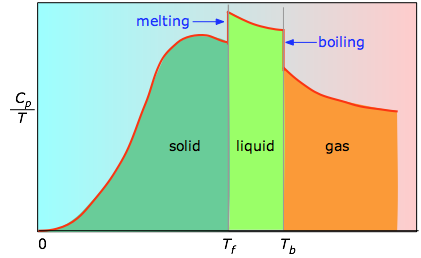Figure $$\PageIndex{3}$$: Heat capacity/temperature as a function of temperature

The area under each section of the plot represents the entropy change associated with heating the substance through an interval ΔT. To this must be added the enthalpies of melting, vaporization, and of any solid-solid phase changes. Values of Cp for temperatures near zero are not measured directly, but can be estimated from quantum theory.Figure $$\PageIndex{4}$$: Molar entropy as a function of temperature

/ Tb are added to obtain the absolute entropy at temperature T. As shown in Figure $$\PageIndex{4}$$ above, the entropy of a substance increases with temperature, and it does so for two reasons:

• As the temperature rises, more microstates become accessible, allowing thermal energy to be more widely dispersed. This is reflected in the gradual increase of entropy with temperature.
• The molecules of solids, liquids, and gases have increasingly greater freedom to move around, facilitating the spreading and sharing of thermal energy. Phase changes are therefore accompanied by massive and discontinuous increase in the entropy.

## Standard Entropies of substances

The standard entropy of a substance is its entropy at 1 atm pressure. The values found in tables are normally those for 298K, and are expressed in units of J K–1 mol–1. The table below shows some typical values for gaseous substances.

Table $$\PageIndex{2}$$: Standard entropies of some gases at 298 K, J K–1 mol–1
He 126 H2 131 CH4 186
Ne 146 N2 192 H2O(g) 187
Ar 155 CO 197 CO2 213
Kr 164 F2 203 C2H6 229
Xe 170 O2 205 n -C3H8 270
Cl2 223 n -C4H10 310

Note especially how the values given in this Table $$\PageIndex{2}$$:illustrate these important points:

• Although the standard internal energies and enthalpies of these substances would be zero, the entropies are not. This is because there is no absolute scale of energy, so we conventionally set the “energies of formation” of elements in their standard states to zero. Entropy, however, measures not energy itself, but its dispersal amongst the various quantum states available to accept it, and these exist even in pure elements.
• It is apparent that entropies generally increase with molecular weight. For the noble gases, this is of course a direct reflection of the principle that translational quantum states are more closely packed in heavier molecules, allowing of them to be occupied.
• The entropies of the diatomic and polyatomic molecules show the additional effects of rotational quantum levels.
Table $$\PageIndex{3}$$: Standard entropies of some solid elements at 298 K, J K–1 mol–1
C(diamond) C(graphite) Fe Pb Na S(rhombic) Si W
2.5 5.7 27.1 51.0 64.9 32.0 18.9 33.5

The entropies of the solid elements are strongly influenced by the manner in which the atoms are bound to one another. The contrast between diamond and graphite is particularly striking; graphite, which is built up of loosely-bound stacks of hexagonal sheets, appears to be more than twice as good at soaking up thermal energy as diamond, in which the carbon atoms are tightly locked into a three-dimensional lattice, thus affording them less opportunity to vibrate around their equilibrium positions. Looking at all the examples in the above table, you will note a general inverse correlation between the hardness of a solid and its entropy. Thus sodium, which can be cut with a knife, has almost twice the entropy of iron; the much greater entropy of lead reflects both its high atomic weight and the relative softness of this metal. These trends are consistent with the oft-expressed principle that the more “disordered” a substance, the greater its entropy.

Table $$\PageIndex{4}$$: Standard entropy of water at 298 K, J K–1 mol–1
solid liquid gas
41 70 186

Gases, which serve as efficient vehicles for spreading thermal energy over a large volume of space, have much higher entropies than condensed phases. Similarly, liquids have higher entropies than solids owing to the multiplicity of ways in which the molecules can interact (that is, store energy.)

### How Entropy depends on Concentration

As a substance becomes more dispersed in space, the thermal energy it carries is also spread over a larger volume, leading to an increase in its entropy. Because entropy, like energy, is an extensive property, a dilute solution of a given substance may well possess a smaller entropy than the same volume of a more concentrated solution, but the entropy per mole of solute (the molar entropy) will of course always increase as the solution becomes more dilute.

For gaseous substances, the volume and pressure are respectively direct and inverse measures of concentration. For an ideal gas that expands at a constant temperature (meaning that it absorbs heat from the surroundings to compensate for the work it does during the expansion), the increase in entropy is given by

$\Delta S = R \ln \left( \dfrac{V_2}{V_1} \right) \label{23.2.4}$

Note: If the gas is allowed to cool during the expansion, the relation becomes more complicated and will best be discussed in a more advanced course.

Because the pressure of a gas is inversely proportional to its volume, we can easily alter the above relation to express the entropy change associated with a change in the pressure of a perfect gas:

$\Delta S = R \ln \left( \dfrac{P_1}{P_2} \right) \label{23.2.5}$

Expressing the entropy change directly in concentrations, we have the similar relation

$\Delta S = R \ln \left( \dfrac{c_1}{c_2} \right) \label{23.2.6}$

Although these equations strictly apply only to perfect gases and cannot be used at all for liquids and solids, it turns out that in a dilute solution, the solute can often be treated as a gas dispersed in the volume of the solution, so the last equation can actually give a fairly accurate value for the entropy of dilution of a solution. We will see later that this has important consequences in determining the equilibrium concentrations in a homogeneous reaction mixture.

### How thermal energy is stored in molecules

Thermal energy is the portion of a molecule's energy that is proportional to its temperature, and thus relates to motion at the molecular scale. What kinds of molecular motions are possible? For monatomic molecules, there is only one: actual movement from one location to another, which we call translation. Since there are three directions in space, all molecules possess three modes of translational motion.

For polyatomic molecules, two additional kinds of motions are possible. One of these is rotation; a linear molecule such as CO2 in which the atoms are all laid out along the x-axis can rotate along the y- and z-axes, while molecules having less symmetry can rotate about all three axes. Thus linear molecules possess two modes of rotational motion, while non-linear ones have three rotational modes. Finally, molecules consisting of two or more atoms can undergo internal vibrations. For freely moving molecules in a gas, the number of vibrational modes or patterns depends on both the number of atoms and the shape of the molecule, and it increases rapidly as the molecule becomes more complicated.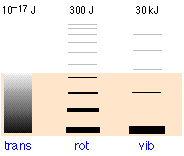Figure $$\PageIndex{5}$$

The relative populations of the quantized translational, rotational and vibrational energy states of a typical diatomic molecule are depicted by the thickness of the lines in this schematic (not-to-scale!) diagram. The colored shading indicates the total thermal energy available at a given temperature. The numbers at the top show order-of-magnitude spacings between adjacent levels. It is readily apparent that virtually all the thermal energy resides in translational states.

Notice the greatly different spacing of the three kinds of energy levels. This is extremely important because it determines the number of energy quanta that a molecule can accept, and, as the following illustration shows, the number of different ways this energy can be distributed amongst the molecules.Figure $$\PageIndex{6}$$

The more closely spaced the quantized energy states of a molecule, the greater will be the number of ways in which a given quantity of thermal energy can be shared amongst a collection of these molecules.

##### Note

The spacing of molecular energy states becomes closer as the mass and number of bonds in the molecule increases, so we can generally say that the more complex the molecule, the greater the density of its energy states.

### Quantum states, microstates, and energy spreading

At the atomic and molecular level, all energy is quantized; each particle possesses discrete states of kinetic energy and is able to accept thermal energy only in packets whose values correspond to the energies of one or more of these states. Polyatomic molecules can store energy in rotational and vibrational motions, and all molecules (even monatomic ones) will possess translational kinetic energy (thermal energy) at all temperatures above absolute zero. The energy difference between adjacent translational states is so minute that translational kinetic energy can be regarded as continuous (non-quantized) for most practical purposes.

The number of ways in which thermal energy can be distributed amongst the allowed states within a collection of molecules is easily calculated from simple statistics, but we will confine ourselves to an example here. Suppose that we have a system consisting of three molecules and three quanta of energy to share among them. We can give all the kinetic energy to any one molecule, leaving the others with none, we can give two units to one molecule and one unit to another, or we can share out the energy equally and give one unit to each molecule. All told, there are ten possible ways of distributing three units of energy among three identical molecules as shown here:Figure $$\PageIndex{7}$$

Each of these ten possibilities represents a distinct microstate that will describe the system at any instant in time. Those microstates that possess identical distributions of energy among the accessible quantum levels (and differ only in which particular molecules occupy the levels) are known as configurations. Because all microstates are equally probable, the probability of any one configuration is proportional to the number of microstates that can produce it. Thus in the system shown above, the configuration labeled ii will be observed 60% of the time, while iii will occur only 10% of the time.

As the number of molecules and the number of quanta increases, the number of accessible microstates grows explosively; if 1000 quanta of energy are shared by 1000 molecules, the number of available microstates will be around 10600— a number that greatly exceeds the number of atoms in the observable universe! The number of possible configurations (as defined above) also increases, but in such a way as to greatly reduce the probability of all but the most probable configurations. Thus for a sample of a gas large enough to be observable under normal conditions, only a single configuration (energy distribution amongst the quantum states) need be considered; even the second-most-probable configuration can be neglected.

The bottom line: any collection of molecules large enough in numbers to have chemical significance will have its therrmal energy distributed over an unimaginably large number of microstates. The number of microstates increases exponentially as more energy states ("configurations" as defined above) become accessible owing to

• Addition of energy quanta (higher temperature),
• Increase in the number of molecules (resulting from dissociation, for example).
• the volume of the system increases (which decreases the spacing between energy states, allowing more of them to be populated at a given temperature.)

### Heat Death: Energy-spreading changes the world

Energy is conserved; if you lift a book off the table, and let it fall, the total amount of energy in the world remains unchanged. All you have done is transferred it from the form in which it was stored within the glucose in your body to your muscles, and then to the book (that is, you did work on the book by moving it up against the earth’s gravitational field). After the book has fallen, this same quantity of energy exists as thermal energy (heat) in the book and table top.

What has changed, however, is the availability of this energy. Once the energy has spread into the huge number of thermal microstates in the warmed objects, the probability of its spontaneously (that is, by chance) becoming un-dispersed is essentially zero. Thus although the energy is still “there”, it is forever beyond utilization or recovery. The profundity of this conclusion was recognized around 1900, when it was first described at the “heat death” of the world. This refers to the fact that every spontaneous process (essentially every change that occurs) is accompanied by the “dilution” of energy. The obvious implication is that all of the molecular-level kinetic energy will be spread out completely, and nothing more will ever happen.

### Why do gases tend to expand, but never contract?

Everybody knows that a gas, if left to itself, will tend to expand and fill the volume within which it is confined completely and uniformly. What “drives” this expansion? At the simplest level it is clear that with more space available, random motions of the individual molecules will inevitably disperse them throughout the space. But as we mentioned above, the allowed energy states that molecules can occupy are spaced more closely in a larger volume than in a smaller one. The larger the volume available to the gas, the greater the number of microstates its thermal energy can occupy. Since all such states within the thermally accessible range of energies are equally probable, the expansion of the gas can be viewed as a consequence of the tendency of thermal energy to be spread and shared as widely as possible. Once this has happened, the probability that this sharing of energy will reverse itself (that is, that the gas will spontaneously contract) is so minute as to be unthinkable.Figure $$\PageIndex{7}$$

Imagine a gas initially confined to one half of a box (Figure $$\PageIndex{7}$$). The barrier is then removed so that it can expand into the full volume of the container. We know that the entropy of the gas will increase as the thermal energy of its molecules spreads into the enlarged space. In terms of the spreading of thermal energy, Figure 23.2.X may be helpful. The tendency of a gas to expand is due to the more closely-spaced thermal energy states in the larger volume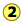.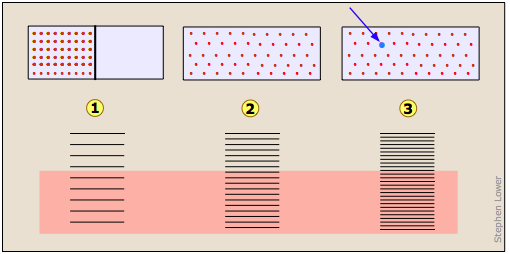Figure $$\PageIndex{8}$$: The pink shading represents the thermally-accessible range of microstates for a given temperature.

### Entropy of mixing and dilution

Mixing and dilution really amount to the same thing, especially for idea gases. Replace the pair of containers shown above with one containing two kinds of molecules in the separate sections (Figure $$\PageIndex{9}$$). When we remove the barrier, the "red" and "blue" molecules will each expand into the space of the other. (Recall Dalton's Law that "each gas is a vacuum to the other gas".) However, notice that although each gas underwent an expansion, the overall process amounts to what we call "mixing".Figure $$\PageIndex{9}$$: Mixing of a gas

What is true for gaseous molecules can, in principle, apply also to solute molecules dissolved in a solvent. But bear in mind that whereas the enthalpy associated with the expansion of a perfect gas is by definition zero, ΔH's of mixing of two liquids or of dissolving a solute in a solvent have finite values which may limit the miscibility of liquids or the solubility of a solute. But what's really dramatic is that when just one molecule of a second gas is introduced into the container (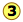in Figure $$\PageIndex{8}$$), an unimaginably huge number of new configurations become possible, greatly increasing the number of microstates that are thermally accessible (as indicated by the pink shading above).

### Why heat flows from hot to cold

Just as gases spontaneously change their volumes from “smaller-to-larger”, the flow of heat from a warmer body to a cooler one always operates in the direction “warmer-to-cooler” because this allows thermal energy to populate a larger number of energy microstates as new ones are made available by bringing the cooler body into contact with the warmer one; in effect, the thermal energy becomes more “diluted”.Figure $$\PageIndex{10}$$: Part a of the figure is a schematic depiction of the thermal energy states in two separated identical bodies at different temperatures (indicated by shading.)

When the bodies are brought into thermal contact (b), thermal energy flows from the higher occupied levels in the warmer object into the unoccupied levels of the cooler one until equal numbers are occupied in both bodies, bringing them to the same temperature. As you might expect, the increase in the amount of energy spreading and sharing is proportional to the amount of heat transferred q, but there is one other factor involved, and that is the temperature at which the transfer occurs. When a quantity of heat q passes into a system at temperature T, the degree of dilution of the thermal energy is given by

$\dfrac{q}{T}$

To understand why we have to divide by the temperature, consider the effect of very large and very small values of Tin the denominator. If the body receiving the heat is initially at a very low temperature, relatively few thermal energy states are initially occupied, so the amount of energy spreading into vacant states can be very great. Conversely, if the temperature is initially large, more thermal energy is already spread around within it, and absorption of the additional energy will have a relatively small effect on the degree of thermal disorder within the body.

### Chemical reactions: why the equilibrium constant depends on the temperature

When a chemical reaction takes place, two kinds of changes relating to thermal energy are involved:

1. The ways that thermal energy can be stored within the reactants will generally be different from those for the products. For example, in the reaction H2→ 2 H, the reactant dihydrogen possesses vibrational and rotational energy states, while the atomic hydrogen in the product has translational states only— but the total number of translational states in two moles of H is twice as great as in one mole of H2. Because of their extremely close spacing, translational states are the only ones that really count at ordinary temperatures, so we can say that thermal energy can become twice as diluted (“spread out”) in the product than in the reactant. If this were the only factor to consider, then dissociation of dihydrogen would always be spontaneous and this molecule would not exist.
2. In order for this dissociation to occur, however, a quantity of thermal energy (heat) qU must be taken up from the surroundings in order to break the H–H bond. In other words, the ground state (the energy at which the manifold of energy states begins) is higher in H, as indicated by the vertical displacement of the right half in each of the four panels below.

In Figure $$\PageIndex{11}$$ are schematic representations of the translational energy levels of the two components H and H2 of the hydrogen dissociation reaction. The shading shows how the relative populations of occupied microstates vary with the temperature, causing the equilibrium composition to change in favor of the dissociation product.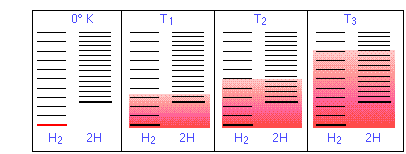Figure $$\PageIndex{11}$$:

The ability of energy to spread into the product molecules is constrained by the availability of sufficient thermal energy to produce these molecules. This is where the temperature comes in. At absolute zero the situation is very simple; no thermal energy is available to bring about dissociation, so the only component present will be dihydrogen.

• As the temperature increases, the number of populated energy states rises, as indicated by the shading in the diagram. At temperature T1, the number of populated states of H2 is greater than that of 2H, so some of the latter will be present in the equilibrium mixture, but only as the minority component.
• At some temperature T2 the numbers of populated states in the two components of the reaction system will be identical, so the equilibrium mixture will contain H2 and “2H” in equal amounts; that is, the mole ratio of H2/H will be 1:2.
• As the temperature rises to T3 and above, we see that the number of energy states that are thermally accessible in the product begins to exceed that for the reactant, thus favoring dissociation.

The result is exactly what the LeChatelier Principle predicts: the equilibrium state for an endothermic reaction is shifted to the right at higher temperatures.

The following table generalizes these relations for the four sign-combinations of ΔH and ΔS. (Note that use of the standard ΔH° and ΔS° values in the example reactions is not strictly correct here, and can yield misleading results when used generally.)

##### Exothermic reaction, ΔS > 0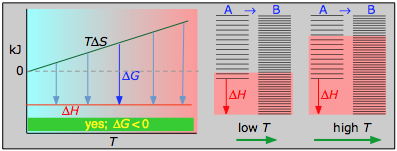This combustion reaction, like most such reactions, is spontaneous at all temperatures. The positive entropy change is due mainly to the greater mass of CO2 molecules compared to those of O2.

< 0

• ΔH° = –46.2 kJ
• ΔS° = –389 J K–1
• ΔG° = –16.4 kJ at 298 K

The decrease in moles of gas in the Haber ammonia synthesis drives the entropy change negative, making the reaction spontaneous only at low temperatures. Thus higher T, which speeds up the reaction, also reduces its extent.

> 0

• ΔH° = 55.3 kJ
• ΔS° = +176 J K–1
• ΔG° = +2.8 kJ at 298 K

Dissociation reactions are typically endothermic with positive entropy change, and are therefore spontaneous at high temperatures.Ultimately, all molecules decompose to their atoms at sufficiently high temperatures.

< 0

• ΔH° = 33.2 kJ
• ΔS° = –249 J K1
• ΔG° = +51.3 kJ at 298 K

This reaction is not spontaneous at any temperature, meaning that its reverse is always spontaneous. But because the reverse reaction is kinetically inhibited, NO2 can exist indefinitely at ordinary temperatures even though it is thermodynamically unstable.

## Phase changes

Everybody knows that the solid is the stable form of a substance at low temperatures, while the gaseous state prevails at high temperatures. Why should this be? The diagram in Figure $$\PageIndex{12}$$ shows that

1. the density of energy states is smallest in the solid and greatest (much, much greater) in the gas, and
2. the ground states of the liquid and gas are offset from that of the previous state by the heats of fusion and vaporization, respectively.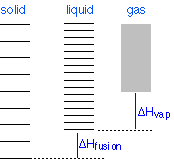Figure $$\PageIndex{12}$$:

Changes of phase involve exchange of energy with the surroundings (whose energy content relative to the system is indicated (with much exaggeration!) by the height of the yellow vertical bars in Figure $$\PageIndex{13}$$. When solid and liquid are in equilibrium (middle section of diagram below), there is sufficient thermal energy (indicated by pink shading) to populate the energy states of both phases. If heat is allowed to flow into the surroundings, it is withdrawn selectively from the more abundantly populated levels of the liquid phase, causing the quantity of this phase to decrease in favor of the solid. The temperature remains constant as the heat of fusion is returned to the system in exact compensation for the heat lost to the surroundings. Finally, after the last trace of liquid has disappeared, the only states remaining are those of the solid. Any further withdrawal of heat results in a temperature drop as the states of the solid become depopulated.Figure $$\PageIndex{13}$$:

## Colligative Properties of Solutions

Vapor pressure lowering, boiling point elevation, freezing point depression and osmosis are well-known phenomena that occur when a non-volatile solute such as sugar or a salt is dissolved in a volatile solvent such as water. All these effects result from “dilution” of the solvent by the added solute, and because of this commonality they are referred to as colligative properties (Lat. co ligare, connected to.) The key role of the solvent concentration is obscured by the greatly-simplified expressions used to calculate the magnitude of these effects, in which only the solute concentration appears. The details of how to carry out these calculations and the many important applications of colligative properties are covered elsewhere. Our purpose here is to offer a more complete explanation of why these phenomena occur.

Basically, these all result from the effect of dilution of the solvent on its entropy, and thus in the increase in the density of energy states of the system in the solution compared to that in the pure liquid. Equilibrium between two phases (liquid-gas for boiling and solid-liquid for freezing) occurs when the energy states in each phase can be populated at equal densities. The temperatures at which this occurs are depicted by the shading.Figure $$\PageIndex{14}$$: Boiling Point Elevation from an entropy perspective

Dilution of the solvent adds new energy states to the liquid, but does not affect the vapor phase. This raises the temperature required to make equal numbers of microstates accessible in the two phases.Figure $$\PageIndex{15}$$: Freezing Point Depression from an entropy perspective

Dilution of the solvent adds new energy states to the liquid, but does not affect the solid phase. This reduces the temperature required to make equal numbers of states accessible in the two phases.

## Effects of pressure on the entropy: Osmotic pressure

When a liquid is subjected to hydrostatic pressure— for example, by an inert, non-dissolving gas that occupies the vapor space above the surface, the vapor pressure of the liquid is raised (Figure $$\PageIndex{16}$$). The pressure acts to compress the liquid very slightly, effectively narrowing the potential energy well in which the individual molecules reside and thus increasing their tendency to escape from the liquid phase. (Because liquids are not very compressible, the effect is quite small; a 100-atm applied pressure will raise the vapor pressure of water at 25°C by only about 2 torr.) In terms of the entropy, we can say that the applied pressure reduces the dimensions of the "box" within which the principal translational motions of the molecules are confined within the liquid, thus reducing the density of energy states in the liquid phase.Figure $$\PageIndex{16}$$:

Applying hydrostatic pressure to a liquid increases the spacing of its microstates, so that the number of energetically accessible states in the gas, although unchanged, is relatively greater— thus increasing the tendency of molecules to escape into the vapor phase. In terms of free energy, the higher pressure raises the free energy of the liquid, but does not affect that of the gas phase.Figure $$\PageIndex{17}$$: Osmotic Pressure from an entropy perspective

This phenomenon can explain osmotic pressure. Osmotic pressure, students must be reminded, is not what drives osmosis, but is rather the hydrostatic pressure that must be applied to the more concentrated solution (more dilute solvent) in order to stop osmotic flow of solvent into the solution. The effect of this pressure $$\Pi$$ is to slightly increase the spacing of solvent energy states on the high-pressure (dilute-solvent) side of the membrane to match that of the pure solvent, restoring osmotic equilibrium.

This page titled 15.2: Entropy Rules is shared under a CC BY 3.0 license and was authored, remixed, and/or curated by Stephen Lower via source content that was edited to the style and standards of the LibreTexts platform; a detailed edit history is available upon request.